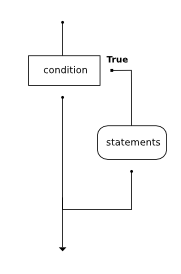# How to Think Like a Computer Scientist: The PreTeXt Interactive Edition

## Section7.5Omitting the else Clause: Unary SelectionAnother form of the if statement is one in which the else clause is omitted entirely. This creates what is sometimes called unary selection. In this case, when the condition evaluates to True, the statements are executed. Otherwise the flow of execution continues to the statement after the body of the if.
What would be printed if the value of x is negative? Try it.

### Checkpoint7.5.1.

What does the following code print?
x = -10
if x < 0:
print("The negative number ",  x, " is not valid here.")
print("This is always printed")

a.
This is always printed

b.
The negative number -10 is not valid here
This is always printed

c.
The negative number -10 is not valid here

• Output a
• Because -10 is less than 0, Python will execute the body of the if-statement here.
• Output b
• Python executes the body of the if-block as well as the statement that follows the if-block.
• Output c
• Python will also execute the statement that follows the if-block (because it is not enclosed in an else-block, but rather just a normal statement).
• It will cause an error because every if must have an else clause.
• It is valid to have an if-block without a corresponding else-block (though you cannot have an else-block without a corresponding if-block).

### Checkpoint7.5.2.

Will the following code cause an error?
x = -10
if x < 0:
print("The negative number ",  x, " is not valid here.")
else:
print(x, " is a positive number")
else:
print("This is always printed")

• No
• Every else-block must have exactly one corresponding if-block. If you want to chain if-else statements together, you must use the else if construct, described in the chained conditionals section.
• Yes
• This will cause an error because the second else-block is not attached to a corresponding if-block.